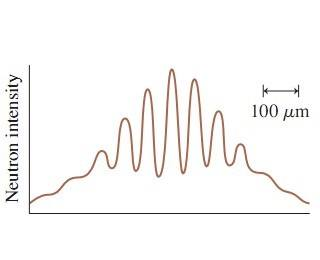# Double-slit experiment: What is the speed of the neutrons?

## Homework Statement

An experiment was performed in which neutrons were shot through two slits spaced 0.10 mm apart and detected 3.5 m behind the slits. The following figure shows the detector output. Notice the 100 μm scale on the figure. To two significant figures, what was the speed of the neutrons?## Homework Equations

Δy = λL/d (spacing between maxima)
λ = h/mv (de Broglie λ)
m= 1.67*10^-27 Kg

## The Attempt at a Solution

The spacing between the maxima appears to be 100*10^-6 m in the figure, so:
(Δy*d)/L = λ = ((100*10^-6 m)*(0.1*10^-3 m))/3.5 m = 2.85714*10^-9 m = de Broglie λ

v = h/(mλ) = (6.626*10^-34 J.s)/((1.67*10^-27 Kg)*(2.85714*10^-9 m) = 138.9 m/s

This answer is incorrect. Can someone please give me a hint as to what I'm doing wrong?

BvU
Homework Helper
spacing between the maxima appears to be 100*10^-6 m in the figure
Could you check that once more for me ?

(oh, and 138.9 is not what I would call 'to two significant figures' ! )

Could you check that once more for me ?

(oh, and 138.9 is not what I would call 'to two significant figures' ! )

Hum, on second look it looks more like (2/3)(100*10^-6m).

Which gives Vneutron = 2.1*10^2 m/s; which is the correct answer! Thanks a lot!

BvU